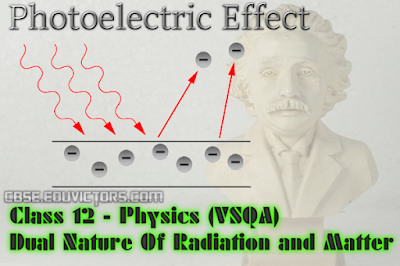Class 12 Physics

## Dual Nature Of Radiation and Matter

Q1: Write the relationship of de-Broglie wavelength λ associated with a particle of mass m in terms of its kinetic energy K.

Answer: Kinetic Energy (K) = p²/2m
where p = momentum and m = mass.
∴ p = √2mK

∴ de-Broglie wavelength λ = h/p = h/ √2mK

Q2: Mention one physical process for the release of an electron from the surface of a metal?

Q3: Name an experiment which shows the wave nature of the electron. Which phenomenon was observed in this experiment using an electron beam?

Answer: Davisson-German experiment shows the wave nature of the electron. Phenomena of constructive interference were observed in the Davisson-Germer experiment.

Q4: What is Thermionic emission?

Answer: It is the phenomenon of emission of electrons from the metal surface when heated suitably.

Q5: Ultraviolet light is incident on two photosensitive materials having work function ϕ₁ and ϕ₂(ϕ₁ >ϕ₂).   In which of the case will K.E. of emitted electrons be greater? Why?

Answer: hv = ϕₒ + KE

If ϕ₁ >ϕ₂, K.E. will be more for the second surface whose work function is less.

Q6: What consideration led de-Broglie to suggest that material particles can also show wave property?

Answer: Nature loves symmetry and matter can be converted into energy and vice- versa, i.e., matter and energy are two forms of the same entity.

Q7: What is photoelectric emission?

Answer: It is the phenomenon of emission of electrons from the surface of the metal when light radiations of suitable frequency fall on it.

Q8: How does the stopping potential apply to a photocell   change  if  the distance    between
the light source and the cathode of the cell are doubled?

Answer: Stopping potential does  not depend on the intensity of the light source which changes
due to the change in distance from the light source.

Q9: If an electron behaves like a wave, what should determine the wavelength and frequency of this wave.

Q10: Is there any difference between light waves and matter waves?

Answer: Yes, the velocity of light waves in a vacuum is constant, whereas the velocity of matter waves in a vacuum depends upon the wavelength of a matter wave.

Q11: What is the Photoelectric effect?

Answer: It is the phenomenon of emission of electrons from the surface of metals when light radiations of suitable frequency fall on them.

Q12: Why is photoelectric emission not possible at all frequencies?

Answer: Photoelectric emission is not possible at all frequencies because below the threshold frequency for a photosensitive surface of different atoms emission is not possible.

Q13: On what factor does the retarding potential of a photocell depend?

Answer: It depends upon the frequency of the incident light.

Q14: What are photons?

Answer: These are the packets of energy (or energy particles) which are emitted by a source of radiation. The photons emitted from a source, travel through space with the same speed c (equal to the speed of light).

Q15: State de-Broglie Hypothesis?

Answer: de Broglie hypothesis states that atomic particles of matter moving with a given velocity, (or
momentum) can display wave-like properties.

Q16: What is Stopping Potential?

Answer: It is that minimum negative potential given to anode in a photocell for which the photoelectric current becomes zero. It is denoted by Vₒ. It is independent of the intensity of the incident light.

Ch 1: Electric Charge and Fields (VSQA)
Electrostatics - Important Theory Questions
Ch 2: Electrostatic Potential and Capacitance (Short Q and A)
Ch 3: Current Electricity - (NCERT Solutions)
Ch 3: Current Electricity - Important Theory Questions
Ch 4: Moving Charges and Magnetism (VSQA)

Chapter - Magnetic Effect Of Current (Theoretical Questions)
Ch: Electromagnetic Induction (EMI) (Important Theory Questions)
Chapter - Electromagnetic Waves (Important Theory Questions)

Ch9 - Ray Optics (Important Theory Questions)
Ch 9 - Ray Optics and Optical Instruments (VSQA)
Light - Reflection From Mirrors (Worksheet)
Ch: Wave Optics (Important Theory Questions)

Ch - Electronic Devices (Revision Sheet)
Ch - Atoms and Nuclei - Cathode Rays and Properties
Electromagnetic Waves - About Electromagnetic Spectrum

Physics Sample Question Paper (2015)
Physics Sample Question Paper and Marking Scheme (2016)
Physics Sample Question Paper (2016-17) (See also Marking Scheme of SQP 2016-17)

Physical Constants
Types of Vectors
Simple Physics Quiz